ISSAC 97 - Meijer G Function Representations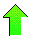Contents News View

 Introduction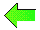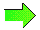Our previous paper "Hypergeometric Function Representations" , presented an algorithm for computing formula representations of the hypergeometric function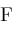defined by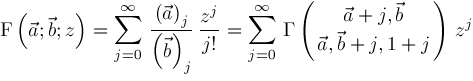where we use notation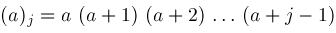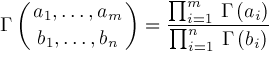For example,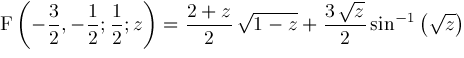is a typical formula representation.  Ability to compute such representations is applicable to integration, differential equations, closed form summation, and difference equations , , 

The Meijer G function,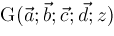, defined in the next section, is a generalization of the hypergeometric function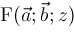.  Every hypergeometric function is a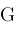function: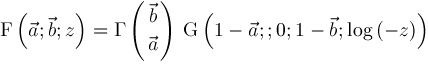However, not everyfunction has a simple representation in terms of hypergeometric functions.  In particular, Bessel functions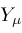and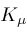(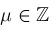), Kelvin functions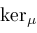and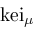(), Whittaker function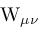(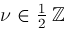), Lommel function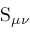(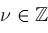), and Legendre function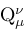() can only be represented byfunctions.

Our new algorithm computes formula representations such as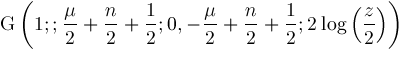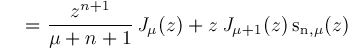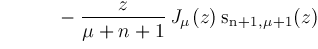An ability to produce such representations is crucially important to the solution of hypergeometric type integrals which appear copiously in various integral tables , , , , used by scientists and mathematicians.

In this paper, we repeat some familiar themes from our previous work , shift operators, contiguity relations, inverse shift operators, suitable origins, accessible origins, proper sequences, and lookup certificates but in a new and different context.  Just the same, the current paper is completely self-contained and will stand on its own.©2004-2021 Planet Quantum Kelly Roach